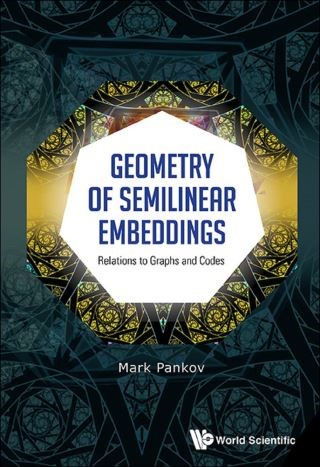## Geometry Of Semilinear Embeddings: Relations To Graphs And Codes• Description
• Author
• Info
• Reviews

### Description

This volume covers semilinear embeddings of vector spaces over division rings and the associated mappings of Grassmannians. In contrast to classical books, we consider a more general class of semilinear mappings and show that this class is important. A large portion of the material will be formulated in terms of graph theory, that is, Grassmann graphs, graph embeddings, and isometric embeddings. In addition, some relations to linear codes will be described. Graduate students and researchers will find this volume to be self-contained with many examples.

Contents:
• Semilinear Mappings:
• Division Rings and Their Homomorphisms
• Vector Spaces Over Division Rings
• Semilinear Mappings
• Semilinear Embeddings
• Mappings of Grassmannians Induced by Semilinear Embeddings
• Kreuzer's Example
• Duality
• Characterization of Strong Semilinear Embeddings
• Projective Geometry and Linear Codes:
• Projective Spaces
• Fundamental Theorem of Projective Geometry
• Proof of Theorem 1.2
• m-independent Subsets in Projective Spaces
• PGL-subsets
• Generalized MacWilliams Theorem
• Linear Codes
• Isometric Embeddings of Grassmann Graphs:
• Graph Theory
• Elementary Properties of Grassmann Graphs
• Embeddings
• Isometric Embeddings
• Proof of Theorem 3.1
• Equivalence of Isometric Embeddings
• Linearly Rigid Isometric Embeddings
• Remarks on Non-isometric Embeddings
• Some Results Related to Chow's Theorem
• Huang's Theorem
• Johnson Graph in Grassmann Graph:
• Johnson Graph
• Isometric Embeddings of Johnson Graphs in Grassmann Graphs
• Proof of Theorem 4.2
• Classification Problem and Relations to Linear Codes
• Characterizations of Apartments in Building Grassmannians
• Characterization of Isometric Embeddings:
• Main Result, Corollaries and Remarks
• Characterization of Distance
• Connectedness of the Apartment Graph
• Intersections of J(n, k)-subsets of Different Types
• Proof of Theorem 5.1
• Semilinear Mappings of Exterior Powers:
• Exterior Powers
• Grassmannians
• Grassmann Codes

Readership: Graduate students and researchers interested in the field of semilinear embeddings.